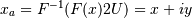# scipy.signal.hilbert¶

scipy.signal.hilbert(x, N=None, axis=-1)[source]

Compute the analytic signal, using the Hilbert transform.

The transformation is done along the last axis by default.

Parameters: x : array_like Signal data. Must be real. N : int, optional Number of Fourier components. Default: x.shape[axis] axis : int, optional Axis along which to do the transformation. Default: -1. xa : ndarray Analytic signal of x, of each 1-D array along axis

Notes

The analytic signal x_a(t) of signal x(t) is:where F is the Fourier transform, U the unit step function, and y the Hilbert transform of x. [R136]

In other words, the negative half of the frequency spectrum is zeroed out, turning the real-valued signal into a complex signal. The Hilbert transformed signal can be obtained from np.imag(hilbert(x)), and the original signal from np.real(hilbert(x)).

References

 [R136] (1, 2) Wikipedia, “Analytic signal”. http://en.wikipedia.org/wiki/Analytic_signal

#### Previous topic

scipy.signal.deconvolve

#### Next topic

scipy.signal.get_window